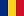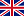A. Puncte pe cerc

A circle is a plane figure bounded by one line, and such that all right lines drawn from a certain point within it to the bounding line, are equal. The bounding line is called its circumference and the point, its centre.” (Euclid, Elemente)

Construiți un cerc care să treacă prin exact 7 puncte care au coordonatele (x,y) numere întregi. Indicați care sunt aceste puncte.

Sursa: IBM Research (problemă indicated de Traian Dajma)

Mai multe informații: Euclid, Elements https://mathcs.clarku.edu/~djoyce/java/elements/toc.htmlA. Points on a circle

A circle is a plane figure bounded by one line, and such that all right lines drawn from a certain point within it to the bounding line, are equal. The bounding line is called its circumference and the point, its centre.” (Euclid, Elemente)

Draw a circle that passes exactly 7 points with coordinates (x,y), both x and y integers. Which are these points?

Source: IBM Research (puzzle indicated by Traian Dajma)